### SAT数学：代数例题 10

2019-09-12 SAT-数学 阅读

# SAT Question Rank: Medium Topic: Plane Geometry, Algebra, Factorizing Quadratics

Below is a question from a recent SAT exam. It is a non-calculator question that would typically take a student 1-2 minutes to complete.

In the xy-plane, the graph of the function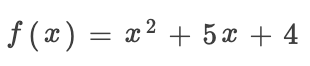has two x-intercepts. What is the distance between the x-intercepts?
(A)1
(B)2
(C)3
(D)4

This curve will the x-axis at the points where y=0. These are the x-intercepts.
So we will set our function equal to 0.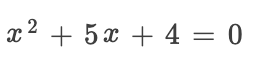Now we have a quadratic equation which we can factorize.
We are looking for 2 numbers which add together to make 5 and multiply together to make 4.
Only 2 numbers satisfy these conditions. +4 and +1.
Therefore, we can place these numbers into double bracket form.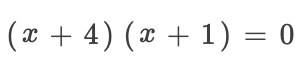We can divide through by each bracket individually to get two solve able equations.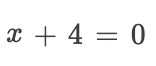and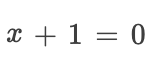So, our solutions are: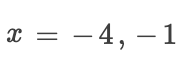Now we just need to find the difference between these numbers.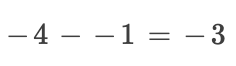As a distance cannot be negative, we use the absolute value of 3.• 地址：401-5811 Cooney Rd, Richmond, BC
• 地址：3030-8171 Ackroyd Rd, Richmond, BC
• 客服热线：604-370-0579 & 604-370-2569
• 联系邮箱：service@forbest.com

• 地址：江苏省无锡市梁溪区清扬路268号三楼
• 客服热线：181-1861-8855

•官方公众号
•加拿大官方客服
•中国官方客服